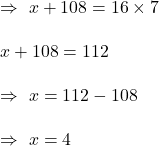## The mean of seven positive integers is 16. When the smallest of these seven integers is removed, the sum of the remaining six integers is 10

Question

The mean of seven positive integers is 16. When the smallest of these seven integers is removed, the sum of the remaining six integers is 108. What is the value of the integer that was removed

in progress 0
6 months 2021-08-09T08:15:06+00:00 1 Answers 15 views 0

1. Answer: The value of the integer that was removed = 4

Step-by-step explanation:

Sum of n values= Mean x n

Given: n = 7 , Mean = 16

Let x= integer removed.

Sum of remaining 6 integers = 6Hence, the value of the integer that was removed = 4